Study P1 Mathematics Shapes 1 - Geniebook# Shapes 1

1. Common Shapes – Circles, Triangles, Rectangles and Squares
2. Properties of Common Shapes
3. Grouping Shapes

## 1. Common Shapes

Some shapes and their examples are given below: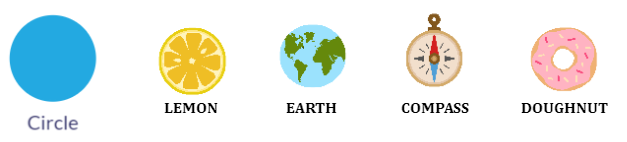Other items in the shape of a circle: ball, clock, coin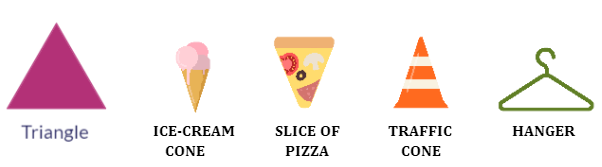Other items in the shape of a triangle: slice of watermelon, flag, roof, christmas tree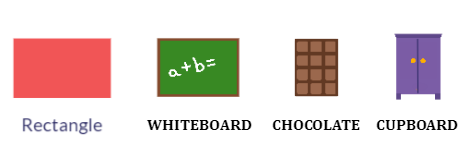Other items in the shape of a rectangle: tissue box, television,, keyboard, shoebox, door, handphone, table, bed, book, red packetOther items in the shape of a square: pizza box, square book

Remember that all the sides of a square are equal, whereas, in rectangles, the opposite sides are equal.

Spot and name the shapes in the picture:• Circle – clock, a picture on the wall, drum
• Square – cushions, a picture on the wall
• Rectangle – three pictures on the wall, television, speakers, books, TV console table
• Triangle – designs on the cushions

Match it!

Amelia was tasked by her mother to buy items of the following shapes from the supermarket. Can you name the items to their correct shapes?Solution:

Circle – watermelon, orange
Triangle – sandwich, a slice of pizza
Rectangle – milk, a bar of chocolate

Question 1:

Name the shape below.1. Circle
2. Square
3. Rectangle
4. Triangle

(4) Triangle

Question 2:

Name the shape below.1. Circle
2. Square
3. Rectangle
4. Triangle

(1) Circle

Question 3:

Name the shape below:1. Circle
2. Square
3. Rectangle
4. Triangle

(2) Square

Question 4:

What is the name of this shape?1. Square
2. Rectangle
3. Triangle
4. Circle

(2) Rectangle

Question 5:

Name the shapes in the figure below.1. circle and rectangle
2. triangle and square
3. circle and triangle
4. rectangle and triangle

(3) circle and triangle

Question 6:

Sam used 2 shapes to draw the robot below. Name the shapes.1. circle and square
2. triangle and square
3. circle and rectangle
4. rectangle and triangle

(3) circle and rectangle

Question 7:

How many squares are there in the figure below?1. 5
2. 6
3. 3
4. 4

(3) 3

Question 8:

How many triangles are there in the figure?1. 5
2. 6
3. 7
4. 8

(4) 8

Question 9:

Which group does not contain a square?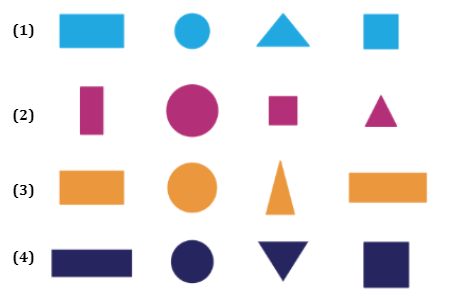(3) Rectangle, circle, triangle, rectangle

Explanation:
1) Rectangle, circle, triangle, square
2) Rectangle, circle, square, triangle
3) Rectangle, circle, triangle, rectangle
4) Rectangle, circle, triangle, square

## 2. Properties Of Common Shapes

Triangle - A triangle has 3 sides and 3 vertices. Vertices are the points where two sides meet.Square – A square has 4 equal sides and 4 vertices.Circle – Circles are round in shape.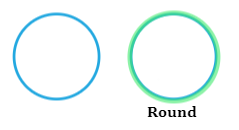Rectangle – A rectangle has 4 sides and 4 vertices.Let’s Try This.

Put the shapes into correct boxes that describe their properties.Solution:

A triangle has 3 vertices.
A square has 4 equal sides.
A circle is round.Question 1:

I have 3 vertices and 3 sides. What shape am I?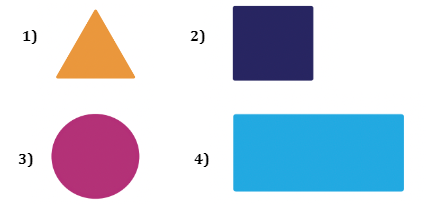(1) Triangle

Explanation:

A triangle has 3 vertices and 3 sides.

Question 2:

I have 4 equal sides. What shape am I?

1. Square
2. Rectangle
3. Triangle
4. Circle

(1) Square

Explanation:

A square has 4 equal sides.

Question 3:

I do not have any vertices. What shape am I?

1. Square
2. Rectangle
3. Triangle
4. Circle

(4) Circle

Explanation:

A circle is round and does not have any vertices.

Question 4:

The figures below are of the same shapes. Is the statement true or false?1. True
2. False

(1) True

Explanation:

They are both triangles.

## 3. Grouping Shapes

We can group shapes based on their:

1. SHAPE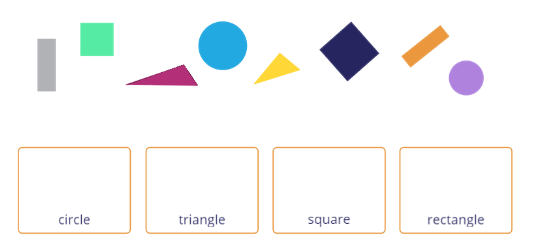They can be grouped as:1. SIZE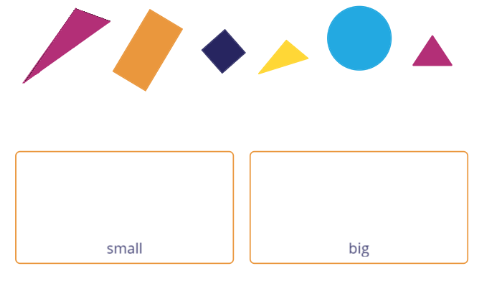They can be grouped as -1. COLOURThey can be grouped as -1. NUMBER OF SIDES AND VERTICESThey can be grouped as –Question 1:

We can group shapes by __________, __________, __________, __________ and __________.

shapes, colours, sizes, sides and vertices.

Question 2:

How are the shapes below being grouped?1. By size
2. By colour
3. By shape
4. By sides and vertices

(1) By size

Explanation:

The shapes in the first box are large in size, whereas the shapes in the second box are small in size.

Question 3:

How are the shapes below being grouped?1. By size
2. By colour
3. By shape
4. By sides and vertices

(2) By colour

Explanation:

The shapes in the first box are blue whereas the shapes in the second box are yellow..

Question 4:

How are the shapes below being grouped?1. By size
2. By colour
3. By shape
4. By sides and vertices

(3) By shape

Explanation:

The shapes in the first box are all triangles, whereas the shapes in the second box are all squares. Therefore, they are grouped by shapes.

Question 5:

Karen cut out some pieces of paper into different shapes and grouped them as shown below. Where should she place the paper below?Group B

Explanation:

Karen should place the paper in Group B as they are the same shape: Square.

Question 6:

How many triangles and circles are there in the figure altogether?1. 5
2. 7
3. 8
4. 9

(4) 9

Explanation:

There are 4 triangles and 5 circles.

$$4 + 5 = 9$$

Question 7:

How many triangles are there in the figure?1. 6
2. 7
3. 8
4. 9

(4) 9

Explanation:

There are 8 triangles in the sun and 1 triangle beside the chimney.

$$8 + 1 = 9$$

## Challenge yourself

Question 1:

How many more squares than triangles are there in the figure?1. 5
2. 7
3. 3
4. 4

(3) 3

Explanation:

There are 7 squares and 4 triangles in the figure.

To find the difference, we need to subtract 4 from 7.

$$7 - 4 = 3$$

Question 2:

How many more circles than rectangles are there in the figure?1. 5
2. 2
3. 7
4. 9

(1) 5

Explanation:

There are 7 circles and 2 rectangles in the figure.

To find the difference, we need to subtract 2 from 7.

$$7 - 2 = 5$$

## Summary: Shapes And Their PropertiesContinue Learning
Numbers To 10 Picture Graphs 1
Shapes 1 Ordinal Numbers
Subtraction Within 20 Addition Within 10
Addition And Subtraction Within 10 Numbers To 20
Addition Within 20 Numbers To 100PrimaryPrimary 1EnglishMaths
Numbers To 10
Picture Graphs 1
Shapes 1
Ordinal Numbers
Subtraction Within 20
Numbers To 20
Numbers To 100Primary 2Primary 3Primary 4Primary 5Primary 6SecondarySecondary 1Secondary 2Secondary 3Secondary 4